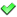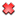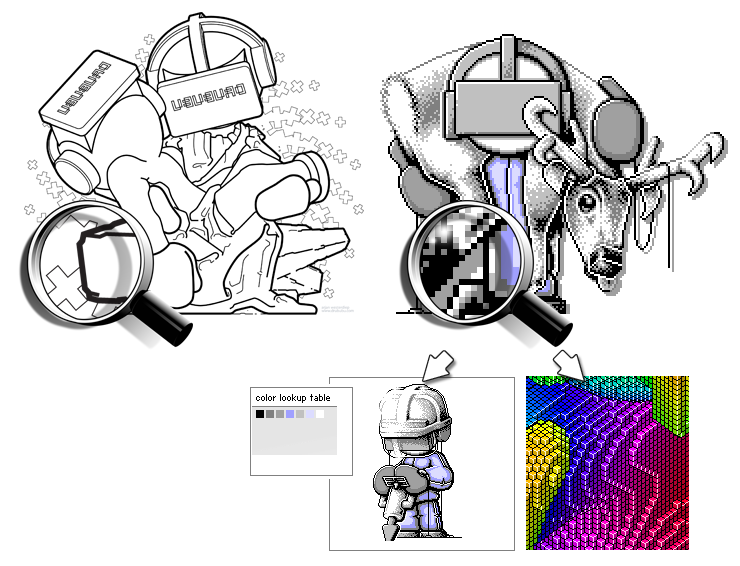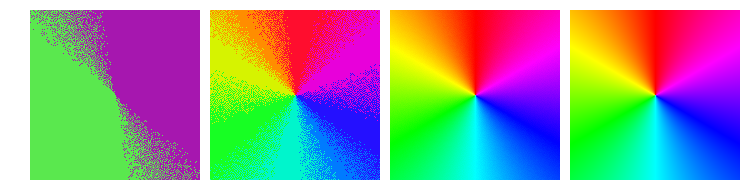# True Colors / Indexed Colors

When you are ready to put your pixel art illustration on the Internet, you have to choose a file format in which to save your work.
In general, you have two types of images in computer graphics: raster graphics, commonly known as bitmap images/bitmap graphics, and vector graphics. Vector graphics use mathematical descriptions of curves.

Pixel art is built on pixels, so you have to use bitmap graphics.

To save your pixel art, you can choose various kinds of file types, such as GIF, PNG, or some other file format that is lossless.

File Format Number of Colors Lossless
PNG unlimitedJPG unlimitedGIF 256File formats like JPG will compress your image by removing small details. The size in kilobytes is reduced by the compression, allowing you to store more images on your hard disk and/or send them more quickly over the Internet. By removing small details, your original image will also lose details. The amount of details that is lost depends on the amount of compression.
The loss of quality makes JPG not a good option in which to save your pixel art illustrations.

Instead of JPG use a file format like GIF or PNG if you want to show your work on the Internet.

Bitmap images can be divided into so-called indexed color (bitmap) images and so-called true color (bitmap) images.Indexed color image with color table and true color image

The decision to use indexed color images or true color images depends on the amount of colors you want to use.

## Colors

Internally a computer uses numbers, represented by so-called 'bits', to describe colors.
The value of a single bit is 0 or 1 (or false or true), nothing else. To designate a number that is not equal to 0 or 1, you need more than one bit.
The binary system uses 2 as its base; our daily decimal system uses 10 as its base. The duodecimal system uses base 12, and is still used for time notation and the months of the year.

## Decimal Numbers

In daily life, we use decimal numbers.

321 (decimal)

The number '1' on the right represent '1' (1 x 1), the '2' represents 20 (2 x 10). '3' represents 300 (3 x 100). The sum of the separate calculations is...321. You probably knew this without realizing that you made the calculation.
The number is read from right to left. The second number is multiplied by 10, the third by 100, etc. The multiplication increases by the power of ten when moved from right to left in the number.
A single number in the decimal system can range from 0 to 9 (base 10 minus one).

## Binairy Numbers

The computer uses internally a binary system. A single binary number - a bit - can be 0 or 1.

110 (binary)

The value of the bit on the right is equal to 0. This 0 is multiplied with 1, just as in the decimal system. The result of the multiplication is, of course, zero.
The second number from the right is 1. Unlike the decimal system that uses 10, 1 is here multiplied by 2. The result of this multiplication is 2. The first and second bits from the right, when combined, add up to 2 ((1 * 2) + (0 * 1)), read from left to right.
The third bit from the right is also 1, but it is multiplied by 4, which results in 4. The binary number 110 therefore represents the decimal value 6 ((1 * 4) + (1 * 2) + (0 * 1)), read from left to right.
By moving from right to left in the binary number, the multiplication increases by the power of two, the base of the binary system.

Bits Minimum Maximum Possibilties Example
1 bit 0 1 2 1 (= 2)
2 bits 0 3 4 11 (= 3)
3 bits 0 7 8 101 (= 5)
4 bits 0 15 16 0001 (= 1)
5 bits 0 31 32 11010 (= 26)
6 bits 0 63 64 111101 (= 61)
7 bits 0 127 128 1001011 (= 75)
8 bits 0 255 256 10000111 (= 135)

To designate numbers within the range of 0 and 255, eight bits are needed.
In the table above, the binary number 10000111 is equal to the decimal number 135 ((1 * 128) + (0 * 64) + (0 * 32) + (0 * 16) + (0 * 8)+(1 * 4) + (1 * 2) + (1 * 1)).

## RGB Colors

A color can be designated by using so-called RGB-values (RGB is the abbreviation of red, green, and blue).
RGB-values range from 0 to 255.

The separate red, green, and blue values can't be used directly to designate a color. A single number is needed, not three.

By using a so-called hexadecimal number (e.g. 0x9B3DD8), a color can be designated by one single number.

The first part '0x' indicates that the number is a hexadecimal number. '9B' is the hexadecimal value of red, '3D' is the hexadecimal value of green, and 'D8' is the hexadecimal value of blue.
A hexadecimal number has 16 as its base. With 16 unique numbers and characters (that represent a number) - 0, 1, 2, 3, 4, 5, 6, 7, 8, 9, A, B, C, D, E and F - every possible integer number can be made.
RGB-values range from 0 to 255. By using two hexadecimal numbers, all numbers between 0 and 255 can be made (16 x 16 possibilties).
In HTML and style sheets, hexadecimal numbers are commonly used to describe colors.
In most programming languages, you are free to use another numerical system, like the octal system (base 8), or the more readable decimal system.

The decimal value of the hexadecimal number 0x9B3DD8 is 10173912 and represents purple (RGB-value 155, 61, 216). This number is not very readable, and it's very difficult to tell which color this number represents, unless you're good at mental calculation.

By using a short cryptic formula, decimal RGB values (e.g. 155, 61, 216) can be substituted for this less readable number, which is the result of the calculation. The computer does the calculation, however, so we don't have to bother about the exact number 10173912.

(155<<16) | (61<<8) | 216;

The hexadecimal number 0x9B3DD8 is the same as the decimal number 10173912.
Both numbers represent a purple color, RGB value 155, 61, 216.
The hexadecimal or decimal number and the separate red, green, and blue values can be designated in the binary system:

```100110110011110111011000 (10173912)
10011011................ red (155)
........00111101........ green (61)
................11011000 blue (216)
```

If you take a good look at the table, you will notice that bits of the red, green, and blue values can be found in the overall binary number. By using so-called bitshifts ('<<') and bitwise inclusive-or's ('|') the red, green, and blue values - 8 bits - are combined into one number.

The red value is shifted 16 bits to the left, the green value is shifted 8 bits to the left, and the blue value is not shifted. To use the formula for a specific RGB color, use `red<<16|green<<8|blue`. Be sure to use integers from 0 to 255 for red, green, and blue.

## Indexed Color Images

Indexed color images use a so-called lookup table with a limited amount of colors. The maximum amount of colors in GIF images is, for example, 256.
Every single pixel in a GIF image uses an index to designate its color. The index points to a specific color in the lookup table.

By changing a color in the lookup table, all pixels with the same index will automatically change color.

By using an index to describe the color of a pixel, it's not necessary to use a much longer RGB-value - a number from 0 to 16.777.216 (256 x 256 x 256 (RGB)). Even small bitmap images have a lot of individual pixels, so the file size is quite small when using a color lookup table.
GIF is a file format that uses a color lookup table, as well as a compression technique to reduce the file size, so that all information is kept to a minimum.
The main disadvantage of indexed color images is their limited palette.
This is only a problem if your pixel art illustration has more colors than the maximum amount of colors in the lookup table of the file format that you are using.

If you want to save an illustration or photo as an indexed color image, and the illustration or photo has more colors than the maximum amount of colors available in the color lookup table, you need to use 'Dithering'.

## True Color Images

Compared to indexed color images, true color images lack a color lookup table.
A pixel doesn't have an index referring to a specific color in the color lookup table. Every pixel has it's own (RGB) color-value, and, depending on the file format, a value for transparency (RGBA).

The RGB color-value is much larger, compared to the index used by an indexed color image. An RGB value contains 24 bits; an RGBA value contains 32 bits.

The main advantage of true color images is the availability of an unlimited amount of colors.

## PNG

The file format PNG - the abbreviation for Portable Network Graphic - supports both color indexed images, grayscale images and true color images, and is ideal for art work that will be placed on the Internet, since, like GIF, it uses lossless compression.
PNG was introduced as a replacement for the older file format GIF; consequently it has some benefits above GIF:

• PNG is patent free (GIF isn't).
• Variable transparency (not just transparent or not like GIF).
• PNG supports true color, grayscale, and indexed color images.
• Better compression than GIF.

GIF still has some benefits/features which PNG has not:

• GIF supports animation.
• GIF is widely supported (PNG as well, but less compared with GIF).

Both file formats support interlacing: an image slowly builds up while the data is being loaded.

Adobe Photoshop and even MS Paint support PNG, as does a lot of other image processing software.

## 1-bit, 8-bits and 24-bits graphics

8-bit (color) graphics are bitmap images with a limited amount of colors. The color per pixel is described using a so-called byte. A byte consists of 8 bits. Each bit is similar to a light switch; it can be on or off, or, more specifically true or false.
Each pixel is represented by one single byte. Its value can range from 0 to 255; 256 possible colors. Each value between 0 and 255 represents a specific color.
8-bits (color) graphics are rapidly vanishing; most of today's graphic hardware uses 24-bits, 32-bits graphics and sometimes even more bits to describe the color value of a single pixel.

Besides 8-bits (color) graphics that use a maximum of 256 colors, there are file formats that use graphics of less than 8 bits. MS Paint allows you to save images as 16 color bitmap images; a maximum of 16 colors can be used.
GIF and PNG allow you to use a (very) limited palette. If your artwork has just 2 colors, say black and white, saving your artwork as a 1-bit graphic is perfectly fine.

Number of Bits Number of Colors File Formats
1-bit 2 GIF, PNG
2-bits 4 GIF, PNG
3-bits 8 GIF, PNG
4-bits 16 GIF, PNG, BMP
5-bits 32 GIF, PNG
6-bits 64 GIF, PNG
7-bits 128 GIF, PNG
8-bits 256 GIF, PNG, BMP
24-bits (= true color) 16.777.216 PNG, JPG, BMP

File formats such as JPG will compress your image by removing small details. In this way, your original image will also lose details. The amount of details lost depends on the degree of compression.
Due to the loss of quality, JPG is not a good option for saving your pixel art illustrations.

Instead of JPG, use a file format like GIF or PNG if you want to display your work on the Internet.from left to right: 1-bit graphic (max. 2 colors), 4-bits graphic (max. 16 colors), 8-bits graphics (max. 256 colors) and 24-bits graphic (max. 16.777.216 colors)

If you save a 24-bits image to an 8-bits image, colors may lose quality and vividness.
An 8-bits image supports a maximum of 256 colors. A 256 color palette is calculated and each color is converted into one of the colors of the palette. The conversion of colors entails a quality loss, which is more or less visible depending on the characteristics of the image.
The loss of quality is especially visible when a 24-bits image is saved as a 1-bits image (see left image); instead of 16,777,216 possible colors (24-bits image) a pixel can have just 2 possible colors (1-bit image).